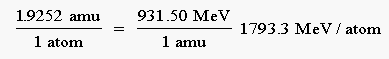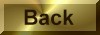Practice Problem 5

Calculate the binding energy of  235U if the mass of this nuclide is 235.0349 amu.

Solution

A neutral  235U  atom contains 92 protons, 92 electrons, and 143 neutrons.   The predicted mass of a  235U  atom is therefore 236.9601 amu, to four significant figures:

[92(1.00728) amu] + [92(0.0005486) amu] + [143(1.00867) amu]  =  236.9601 amu

total mass = 236.9601 amu

To calculate the mass defect for this nuclide, we subtract the observed mass from the predicted mass:

236.9601 amu - 235.0349 amu = 1.9252 amu

mass defect = 1.9252 amu

Using the conversion factor that relates the binding energy to the mass defect, we obtain a binding energy for 235U of 1793.3 MeV per atom: